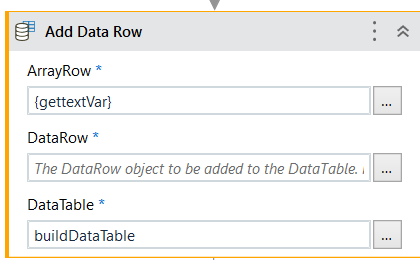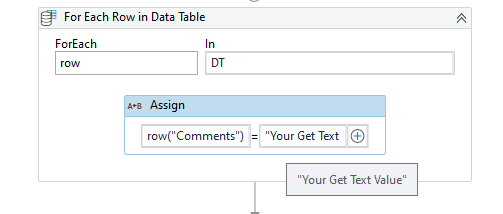# Get text in loop ans string concatenation

I use get text in a loop and i print the output in an excel. I want the output to look like under the column name called comments. How do i achieve this?
UT1:(value of get text)
UT2:(value of get text)
UT3:(value of get text)

Check out the skeleton of the workflow

LoopGetText.xaml (9.3 KB)

Regards
Gokul

@Gokul001 Thank you for the quick response to be precise i want my output to look like this -

For the value in comments column we use get text.

1. build a data table with column comments.

2. in for each loop use the get text activity with dynamic variable.

3. pass the variable of get text in add data row like4. outside the loop use a write range activity and and and pass here build data table varialbe.

Hi @RACHEL_PAUL ,

Not sure what methods you have followed. One of the methods would be to use `Write Cell` Activity in a `For Each` Loop, for this approach you would need to know the position of the Column “Comments” in the Excel, say `A1` or `B1` or `C1` etc…

We could achieve it in a below manner :

Here, “C” is used considering that the Column `Comments` is in that position.

If a Datatable Approach is used and we are using `For Each Row` Activity to loop through Datatable, then we could consider it as an Update operation on Datatable.

Using an `Assign` Activity we should be able to Update the Comments for each of the Rows like shown below :You can try with @supermanPunch suggestion

Use Assign activity

``````CurrentRow("Comment") = GetText Variable
``````

Regards
Gokul

i am using studio version 2021.10.5, i do not have a option called index in properties of the write cell activity

@RACHEL_PAUL , The `Index` Property is present in the `For Each` Activity.

Found it, Thank you. Regarding the process i just use a read range and at the end of the loop i use a write range so i cannot use the write cell in the for each loop as i requires excel scope

We assume a `For Each Row` Activity is used, if so then an `Assign` Activity should be implemented like suggested above for updating the column value `Comments`.

Also Make sure to keep the `Write Range` Activity outside the `For Each Row` Loop.

Were you able to get your required outcome using this method ?

We have Workbook `Write Cell` Activity as well, which does not require `Excel Application Scope`.

Main (5).xaml (21.3 KB)
Input.xlsx (9.5 KB)
Sample xaml kindly have a look.

What is the purpose of `Get Text` Activity in the workflow ?

If you simply want to get the Data submitted by users in `Input Dialog`, then there is no need for `Get Text` Activity, as the result from `Input Dialog` will contain the value entered into Input Dialog.

This is just a Sample workflow hence the purpose is not determined but in my real time project i am facing a similar situation hence the get text activity.

If that is the case, then the Sequence of operations seems to be correct.

Are you facing issues in the real time scenario ?

Im having trouble writing the ouput for comments column as
UT1:(value of get text)
UT2:(value of get text)
UT3:(valhe of get text)

@RACHEL_PAUL , What is the Current output format and what is the Expected format ?

Also, If you Let us know What is the Comment value data then it would be helpful for us to understand and provide a better suggestion.

For the current value of comments each letter of the sentence is an id. For the expected one i want it to be
UT1: ( value of get text)
UT2: (value of get text)

They can be separated by a comma but new line in the same cell also is preferred.

Also lets assume there is an appliaction which generates comments automatically by entering the name subect and grade in the application for each UT and captures the value comments.

How do i give each value a index?